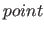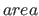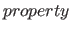Next: Points Up: Elements of the Fib Previous: Elements of the Fib   Contents   Index

## Vectors

Vectors are used for providing numerical values. There are different types of vectors (e. g. position vectors, range vectors or property vectors for RGB colors). The type of a vector (and optionally information in the root-element) gives the domain and the number of the elements that it contains. Each vector element is either a number or a variable, which is set in the Fib object above the Fib element of the vector.

If the value of an evalued element is outside of its domain (the domain for the vector element), this value is rounded to the nearest value that lies in the domain. If an element of the vector is not existing, it is evaluated as the zero value of its domain.

Vectors of one type are only used in a very specific type of Fib element.

A listing of the possible vector typs can be found in Table 1 .

Table 1: Vector typs
 vector description used in Fib element number of elements typ of elements position the position of a pointany (a vectors can always contain just 0 elements) numbers area contains the borders of an area (inclusive the borders)2 integers property vector (there are more than one type) defines a value of a propertyany numbersNext: Points Up: Elements of the Fib Previous: Elements of the Fib   Contents   Index
Betti Österholz 2013-02-13